•5星
7.22MB dhy1688 2021-07-20 15:09:43
•5星
62.23MB weixin_43532002 2021-04-03 15:13:29
•5星
5.5MB u013883025 2021-08-14 19:10:22
•5星
7.2MB cc605523 2020-11-13 15:19:56
•5星
7.27MB cc605523 2020-11-12 18:16:03
•5星
487KB cc605523 2020-11-12 16:58:37
•5星
226KB cc605523 2020-11-12 18:17:07
•5星
35.2MB weixin_43911798 2021-03-25 21:17:48
•5星
466KB cc605523 2020-11-12 18:17:48
•5星
4.45MB cc605523 2020-11-12 16:58:03
• 6.68MB hongweili25 2020-04-20 06:49:05
• 270KB weixin_38687648 2020-06-29 01:57:24
• 5.76MB yuju33676 2021-01-02 18:03:45
• 306B weixin_39662077 2018-07-17 06:30:18
• 机床加工坐标系——定位问题的一些认识 人工智能

当然这个问题主要是针对端程序建立了不同的加工坐标系的基础上，我们都知道一次加工个程序段机床一般都是一次加工定位的，那么不同段的数控程序都是以一个坐标系下的XYZAB值运动还是以各自加工坐标系下的XYZ值...

关于机床加工坐标系的一些认识

前言

在之前的学习过程中，内心一直有个疑惑，UG CAM生成的程序到底是以哪个为基准坐标系的呢？当然这个问题主要是针对多端程序建立了不同的加工坐标系的基础上，我们都知道一次加工的多个程序段机床一般都是一次加工定位的，那么不同段的数控程序都是以一个坐标系下的XYZAB值运动还是以各自加工坐标系下的XYZ值运动呢？我们针对这个问题进行一个探讨!

测试流程：

测试方法

我们先用UG画一个加工图，分两个面进行加工，一个水平面，一个垂直面，坐标系情况如下：（主坐标系为侧面，及Z轴朝外，X轴朝右，Y轴朝上，同机床坐标系不一致）
① 我们设定一个基础坐标系，另一个在此坐标系下旋转
② 我们设定两个坐标系，互相无关联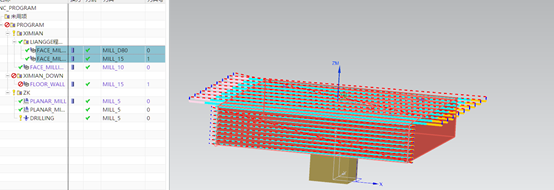两段程序图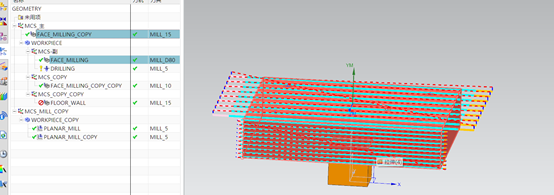坐标系建立情况①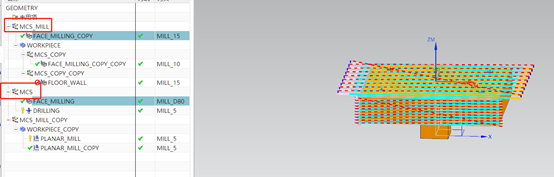坐标系建立情况②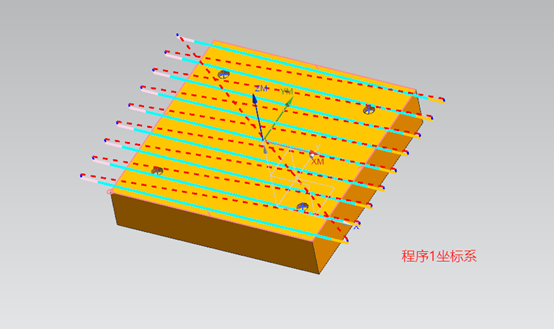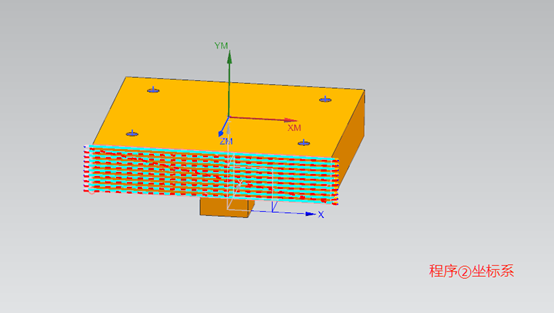测试程序过程测试①：

加工程序一：G0 A270 B0（%因为我们的相对坐标系相对于主坐标系是逆时针旋转90的结果，所以需要相对基础坐标系进行旋转） → G0 X330 Y-232 Z13（快速定位）→ G1 Z2.5 X290（逼近）→X-290（切削）…以当前坐标系定位
加工程序二：G0 A0.0 B0.0（%同机床坐标系方向一致）→G0 X265. Y-97 Z263 →Z255.5
→G1 Z252.5 X257.5→X-257.5（切削）→X-265… 重新以当前坐标系定位，但经过坐标旋转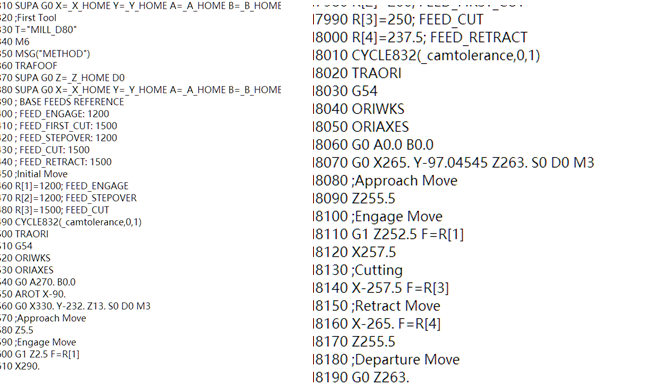测试②：
加工程序一：G0 A0 B0（%默认当前坐标系为定位主坐标系） → G0 X330 Y-232 Z13（快速定位）→ G1 Z2.5 X290（逼近）→X-290（切削）…以当前坐标系定位
加工程序二：G0 A0.0 B0.0（%同机床坐标系方向一致）→G0 X265. Y-97 Z263 →Z255.5
→G1 Z252.5 X257.5→X-257.5（切削）→X-265…以当前坐标系定位，未经过坐标旋转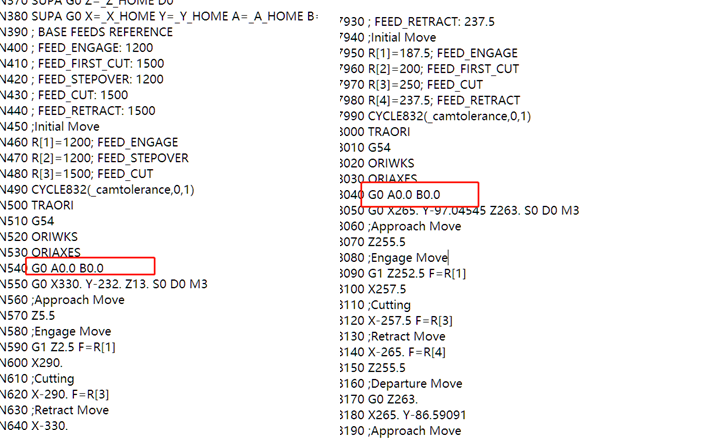测试程序分析及结论

分析

从上述两程序对比，显然可见***数控加工程序是以加工坐标系进行定位的***，其中测试一中一坐标系为主坐标系，另一坐标系为局部坐标系（即依据主坐标系进行旋转），如下图，故得到的程序中***存在局部坐标系的旋转变换，只需对主坐标系进行定位即可***。而测试二中，两坐标系均为主坐标系，无相关联，在加工过程中如果只进行一次定位，显然程序二会定位不当，出现问题。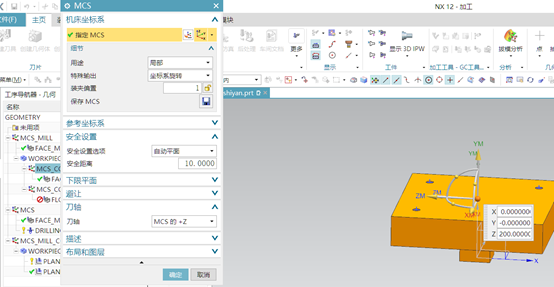结论

以上，可以确认，UG输出的数控程序代码以建立的加工坐标系为基准，同实际机床坐标系并无关联，需要进行对刀定位后机床进行自动计算位置关系。在多个加工坐标系中建立的加工程序代码均是以所在加工坐标系关系进行输出。

展开全文jiachin 2021-03-11 08:58:49
• 相机参数和相机模型各个坐标系(世界坐标系、相机坐标系、图像坐标系、像素坐标系之间变换） 相机参数 世界坐标系 图像坐标系 像素坐标系

相机坐标系(camera coordinate system)：在相机上建立的坐标系，为了从相机的角度描述物体位置而定义，作为沟通世界坐标系和图像/像素坐标系的中间一环。单位为m。 图像坐标系(image coordinate...

坐标系变换

1. 几个坐标系：

• 世界坐标系(world coordinate system)：
用户定义的三维世界的坐标系，为了描述目标物在真实世界里的位置而被引入。单位为m。
• 相机坐标系(camera coordinate system)：在相机上建立的坐标系，为了从相机的角度描述物体位置而定义，作为沟通世界坐标系和图像/像素坐标系的中间一环。单位为m。
• 图像坐标系(image coordinate system)：为了描述成像过程中物体从相机坐标系到图像坐标系的投影透射关系而引入，方便进一步得到像素坐标系下的坐标。 单位为m。
• 像素坐标系(pixel coordinate system)：为了描述物体成像后的像点在数字图像上（相片）的坐标而引入，是我们真正从相机内读取到的信息所在的坐标系。单位为个（像素数目）。

2.相机参数

16个单目相机的参数：

• 4个内部参数（只与相机有关）：
f x , f y , u 0 , v 0 , ( 1 / d x 、 1 / d y 、 γ 、 u 0 、 v 0 、 f ) fx,fy,u0,v0,(1/dx、1/dy、\gamma 、u0、v0、f )
实际其 f x = F ∗ S x fx = F*S_x ，其中的 F F 就是焦距(上面的 f f ).Sx是像素/每毫米(即上面的 1 / d x 1/dx )，其是最后面图里的后两个矩阵进行先相乘，得出的，则把它看成整体，就相当于4个内参。把 γ \gamma 等于零，实际上也是六个.

• 5个畸变参数D：
k 1 , k 2 , k 3 k1,k2,k3 径向畸变系数;
p 1 , p 2 p1,p2 是切向畸变系数;
径向畸变：产生原因是光线在远离透镜中心的地方比靠近中心的地方更加弯曲,径向畸变主要包含桶形畸变和枕形畸变两种。
切向畸变：产生的原因透镜不完全平行于图像平面，这种现象发生于成像仪被粘贴在摄像机的时候。

• 6个外部参数(取决于相机在世界中的位置):
3个旋转参数 R R ;
3个平移参数 T T

3. 坐标系之间的转换

3.1 整体公式

Z c [ u v 1 ] = [ 1 d x γ c x 0 1 d y c y 0 0 1 ] [ f 0 0 0 f 0 0 0 1 ] [ R t 0 T 1 ] [ X w Y w Z w 1 ] = [ f x 0 c x 0 f y c y 0 0 1 ] [ R t 0 T 1 ] [ X w Y w Z w 1 ] Z_c\left[\begin{array}{c}{u } \\ {v} \\ {1}\end{array}\right] =\left[\begin{array}{ccc}{\frac{1}{dx}} & {\gamma} & {c_x} \\ {0} & {\frac{1}{dy}} & {c_y} \\ {0} & {0} & {1}\end{array}\right] \left[\begin{array}{ccc}{f} & {0} & {0} \\ {0} & {f} & {0} \\ {0} & {0} & {1}\end{array}\right] \left[\begin{array}{cc}{\boldsymbol{R}} & {\boldsymbol{t}} \\ {\boldsymbol{0}^{T}} & {1}\end{array}\right] \left[\begin{array}{c}{X_w } \\ {Y_w} \\ {Z_w} \\{1}\end{array}\right]=\left[\begin{array}{ccc}{f_x} & {0} & {c_x} \\ {0} & {f_y} & {c_y} \\ {0} & {0} & {1}\end{array}\right] \left[\begin{array}{cc}{\boldsymbol{R}} & {\boldsymbol{t}} \\ {\boldsymbol{0}^{T}} & {1}\end{array}\right] \left[\begin{array}{c}{X_w } \\ {Y_w} \\ {Z_w} \\{1}\end{array}\right]

3.2 世界坐标系->相机坐标系：

刚体变换(regidbody motion):
三维空间中，当物体不发生形变时，对一个几何物体作旋转 R R ， 平移 T T 的运动，称之为刚体变换。世界坐标系到相机坐标系的变化就是刚体变换，又因为 R R T T 与相机无关，所有又称其为相机外参。
首先考虑旋转。我们设某个单位正交基 ( e 1 ; e 2 ; e 3 ) (e_1; e_2; e_3) 经过一次旋转，变成了 ( e 1 ′ ; e 2 ′ ; e 3 ′ ) (e_1′ ; e_2′ ; e_3′ ) 。那么，对于同一个向量 a（注意该向量并没有随着坐标系的旋转而发生运动），它在两个坐标系下的坐标为 [ a 1 ; a 2 ; a 3 ] T [a_1; a_2; a_3]^T [ a 1 ′ ; a 2 ′ ; a 3 ′ ] T [a_1′ ; a_2′ ; a_3′ ]^T

根据坐标的定义，有

[ e 1 , e 2 , e 3 ] [ a 1 a 2 a 3 ] = [ e 1 ′ , e 2 ′ , e 3 ′ ] [ a 1 ′ a 2 ′ a 3 ′ ] \left[e_{1}, e_{2}, e_{3}\right]\left[\begin{array}{l}{a_{1}} \\ {a_{2}} \\ {a_{3}}\end{array}\right]=\left[e_{1}^{\prime}, e_{2}^{\prime}, e_{3}^{\prime}\right]\left[\begin{array}{c}{a_{1}^{\prime}} \\ {a_{2}^{\prime}} \\ {a_{3}^{\prime}}\end{array}\right]
左右同事乘

[ e 1 T e 2 T e 3 T ] \left[\begin{array}{c}{e_{1}^{T}} \\ {e_{2}^{T}} \\ {e_{3}^{T}}\end{array}\right]
，得到

[ a 1 a 2 a 3 ] = [ e 1 T e 1 ′ e 1 T e 2 ′ e 1 T e 3 ′ e 2 T e 1 ′ e 2 T e 2 ′ e 2 T e 3 ′ e 3 T e 1 ′ e 3 T e 2 ′ e 3 T e 3 ′ ] [ a 1 ′ a 2 ′ a 3 ′ ] ≜ R a ′ \left[\begin{array}{l}{a_{1}} \\ {a_{2}} \\ {a_{3}}\end{array}\right]=\left[\begin{array}{lll}{e_{1}^{T} e_{1}^{\prime}} & {e_{1}^{T} e_{2}^{\prime}} & {e_{1}^{T} e_{3}^{\prime}} \\ {e_{2}^{T} e_{1}^{\prime}} & {e_{2}^{T} e_{2}^{\prime}} & {e_{2}^{T} e_{3}^{\prime}} \\ {e_{3}^{T} e_{1}^{\prime}} & {e_{3}^{T} e_{2}^{\prime}} & {e_{3}^{T} e_{3}^{\prime}}\end{array}\right]\left[\begin{array}{c}{a_{1}^{\prime}} \\ {a_{2}^{\prime}} \\ {a_{3}^{\prime}}\end{array}\right] \triangleq R a^{\prime}
中间的矩阵 R R 由两组基之间的内积组成，刻画了旋转前后同一个向量的坐标变换关系。只要旋转是一样的，那么这个矩阵也是一样的。可以说，矩阵 R R 描述了旋转本身。因此它又称为旋转矩阵。
旋转矩阵有一些特别的性质：是一个行列式为 1 的正交矩阵
可以把旋转矩阵的集合定义如下：

S O ( n ) = { R ∈ R n × n ∣ R R T = I , det ⁡ ( R ) = 1 } SO(n)=\left\{\boldsymbol{R} \in \mathbb{R}^{n \times n} | \boldsymbol{R} \boldsymbol{R}^{T}=\boldsymbol{I}, \operatorname{det}(\boldsymbol{R})=1\right\}
n n 代表坐标轴个数，比如 S O ( 3 ) SO(3) 就代表包含 x ， y ， z x，y，z 三个坐标轴的坐标系。
在欧氏变换中，除了旋转之外还有一个平移。考虑世界坐标系中的向量 $a$，经过一次
旋转 R R 和一次平移 t t 后，得到了 a ′ a′ ，那么把旋转和平移合到一起，有：

a ′ = R a + t \boldsymbol{a}^{\prime}=\boldsymbol{R} \boldsymbol{a}+\boldsymbol{t}
其实表示为坐标就是

[ X c Y c Z c ] = [ r 00 r 01 r 02 r 10 r 11 r 12 r 20 r 21 r 22 ] [ X Y Z ] + [ T x T y T z ] \left[\begin{array}{c}{X_{\mathrm{c}}} \\ {Y_{\mathrm{c}}} \\ {Z_{\mathrm{c}}}\end{array}\right]=\left[\begin{array}{ccc}{r_{00}} & {r_{01}} & {r_{02}} \\ {r_{10}} & {r_{11}} & {r_{12}} \\ {r_{20}} & {r_{21}} & {r_{22}}\end{array}\right]\left[\begin{array}{c}{X} \\ {Y} \\ {Z}\end{array}\right]+\left[\begin{array}{c}{T_{x}} \\ {T_{y}} \\ {T_{z}}\end{array}\right]
上面变换关系不是一个线性关系。假设我们进行了两次变换： R 1 ; t 1 R1; t1 R 2 ; t 2 R2; t2 ，满足：
b = R 1 a + t 1 , c = R 2 b + t 2 \boldsymbol{b}=\boldsymbol{R}_{1} \boldsymbol{a}+\boldsymbol{t}_{1}, \quad \boldsymbol{c}=\boldsymbol{R}_{2} \boldsymbol{b}+\boldsymbol{t}_{2} 合并为 c = R 2 ( R 1 a + t 1 ) + t 2 c=R_{2}\left(R_{1} a+t_{1}\right)+t_{2} ，这样当变化次数特别多的时候很复杂。
所以一般在三维向量的末尾添加一个1，表示为齐次坐标的形式

[ a ′ 1 ] = [ R t 0 T 1 ] [ a 1 ] ≜ T [ a 1 ] \left[\begin{array}{c}{a^{\prime}} \\ {1}\end{array}\right]=\left[\begin{array}{cc}{\boldsymbol{R}} & {\boldsymbol{t}} \\ {\boldsymbol{0}^{T}} & {1}\end{array}\right]\left[\begin{array}{l}{\boldsymbol{a}} \\ {1}\end{array}\right] \triangleq \boldsymbol{T}\left[\begin{array}{l}{\boldsymbol{a}} \\ {1}\end{array}\right]
对于这个四维向量，我们可以把旋转和平移写在一个矩阵里面，使得整个关系变成了线性关系。该式中，矩阵 T T 称为变换矩阵（Transform Matrix） 。
相机坐标系和世界坐标系的坐标原点之间的距离，受 x , y , z x,y,z 三个方向上的分量共同控制，所以具有三个自由度。 R R 其实是分别绕 X , Y , Z X, Y, Z 三轴旋转的效果之和.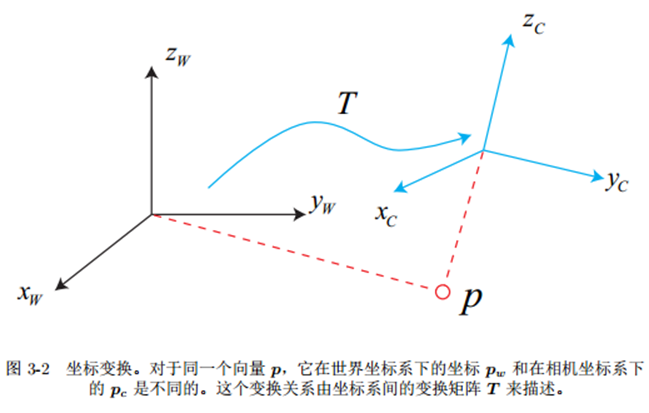绕z轴旋转如右图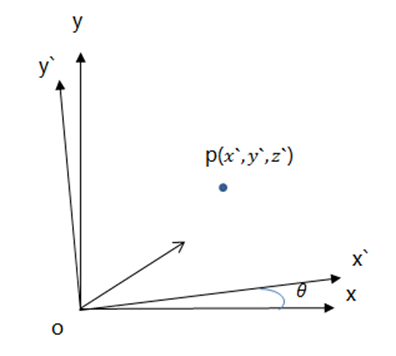绕 z 旋 转 矩 阵 ： [ X c Y c Z c ] = [ 1 0 0 0 cos ⁡ θ sin ⁡ θ 0 − sin ⁡ θ cos ⁡ θ ] [ X Y Z ] 绕z旋转矩阵： \left[\begin{array}{l}{X_{c}} \\ {Y_{c}} \\ {Z_{c}}\end{array}\right]=\left[\begin{array}{ccc}{1} & {0} & {0} \\ {0} & {\cos \theta} & {\sin \theta} \\ {0} & {-\sin \theta} & {\cos \theta}\end{array}\right]\left[\begin{array}{l}{X} \\ {Y} \\ {Z}\end{array}\right]
绕 x 旋 转 矩 阵 ： [ X c Y c Z c ] = [ cos ⁡ θ 0 − sin ⁡ θ 0 1 + 1 1 0 sin ⁡ θ 0 cos ⁡ θ ] [ X Y Z ] 绕x旋转矩阵： \left[\begin{array}{c}{X_{c}} \\ {Y_{c}} \\ {Z_{c}}\end{array}\right]=\left[\begin{array}{ccc}{\cos \theta} & {0} & {-\sin \theta} \\ {0_{1}+1} & {1} & {0} \\ {\sin \theta} & {0} & {\cos \theta}\end{array}\right]\left[\begin{array}{c}{X} \\ {Y} \\ {Z}\end{array}\right]
绕 y 旋 转 矩 阵 ： [ X c Y c Z c ] = [ cos ⁡ θ sin ⁡ θ 0 − sin ⁡ θ cos ⁡ θ 0 0 0 1 ] [ X Y Z ] 绕y旋转矩阵： \left[\begin{array}{l}{X_{\mathrm{c}}} \\ {Y_{\mathrm{c}}} \\ {Z_{\mathrm{c}}}\end{array}\right]=\left[\begin{array}{ccc}{\cos \theta} & {\sin \theta} & {0} \\ {-\sin \theta} & {\cos \theta} & {0} \\ {0} & {0} & {1}\end{array}\right]\left[\begin{array}{c}{X} \\ {Y} \\ {Z}\end{array}\right]
以上分别为绕 x , y , z x, y, z 轴旋转的矩阵，所以旋转矩阵为 R = R x ∗ R y ∗ R z R=R_x*R_y * R_z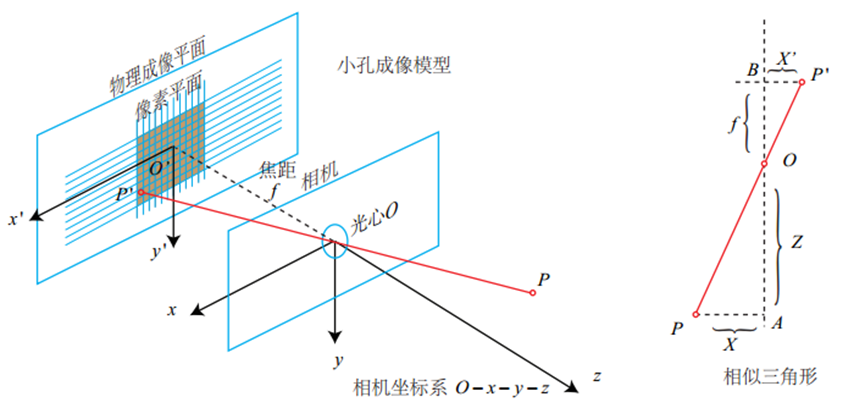O − x − y − z O-x-y-z 为相机坐标系， z z 轴指向相机前方， O O 为摄像机的光心，也是针孔模型中的针孔。

3.3 相机坐标系->图像坐标系：

现实世界的空间点 P P ，经过小孔 O O 投影之后，落在物理成像平面 O ′ − x ′ − y ′ O′-x′-y′ 上，成像点为 P ′ P′ 。设 P P 的坐标为 [ X ; Y ; Z ] T [X; Y; Z]^T P ′ P′ [ X ′ ; Y ′ ; Z ′ ] T [X′; Y ′; Z′]^T ，并且设物理成像平面到小孔的距离为 f f （焦距）。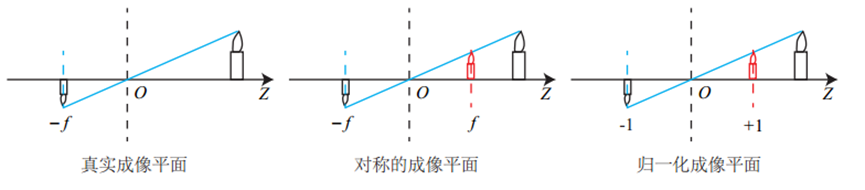我们平时处理一般会用归一化成像平面，把成像位置转换到与它对称的红色位置，这样比较容易描述各个坐标系之间关系。

根据三角形相似关系，有 Z f = − X X ′ = − Y Y ′ \frac{Z}{f}=- \frac{X}{X'}=- \frac{Y}{Y'} 为了方便，一般去掉负号。
整理后：

X ′ = f X Z X′ = \frac{fX}{Z}
Y ′ = f Y Z Y′ = \frac{fY}{Z}

3.4 图像坐标系->像素坐标系：

因为相机最后得到的是像素，所以在成像平面上对像进行采样和量化，把图像坐标系的点转化到一个像素平面 o − u − v o-u-v 。像素坐标系与图像坐标系处于同一平面，像素坐标系原点为左上角，图像坐标系原点为中心。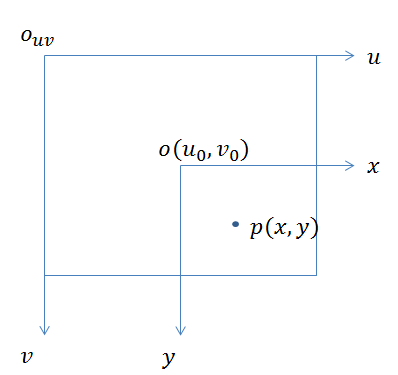像素坐标系‹通常的定义方式是：原点 o ′ o′ 位于图像的左上角， u u 轴向右与 x x 轴平行， v v
轴向下与 y y 轴平行。像素坐标系与成像平面之间，相差了一个缩放和一个原点的平移。
设像素坐标在 u u 轴上缩放了 α α 倍，在 v 上 缩 放 了 上缩放了 β$倍。同时，原点平移了 [ c x ; c y ] T [cx;cy]^T 。那么， P ′ P′ 的坐标与像素坐标 [ u ; v ] T [u;v]^T 的关系为： { u = α X ′ + c x = 1 d x X ′ + c x v = β X ′ + c y = 1 d y Y ′ + c y \begin{cases} u = α X' + c_x = \frac{1}{dx} X' + c_x \\ v = βX' + c_y = \frac{1}{dy} Y' + c_y \end{cases} 1 d x \frac{1}{dx} , 1 d y \frac{1}{dy} 分别为在 x x , y y 方向上每个像素的物理尺寸,把 α f αf 合并为 f x f_x β f βf 合并为 f y f_y 得到： { u = f x X Z + c x v = f y Y Z + c y \begin{cases} u = f_x\frac{X}{Z}+ c_x \\ v = f_y\frac{Y}{Z} + c_y \end{cases}$f $的单位为米， α ; β α; β 的单位为像素每米，所以 f x ; f y f_x; f_y 的单位为像 素。 把该式写成矩阵形式，会更加简洁，不过左侧需要用到齐次坐标, γ \gamma x , y x, y 不垂直时的扭曲系数，一般为0： [ u v 1 ] = 1 Z [ f x γ c x 0 f y c y 0 0 1 ] [ X Y Z ] = 1 Z K P \left[\begin{array}{c}{u } \\ {v} \\ {1}\end{array}\right] = \frac{1}{Z} \left[\begin{array}{ccc}{f_x} & {\gamma} & {c_x} \\ {0} & {f_y} & {c_y} \\ {0} & {0} & {1}\end{array}\right] \left[\begin{array}{c}{X } \\ {Y} \\ {Z}\end{array}\right] = \frac{1}{Z} KP 习惯把Z移动到左面： Z [ u v 1 ] = [ f x 0 c x 0 f y c y 0 0 1 ] [ X Y Z ] = K P Z\left[\begin{array}{c}{u } \\ {v} \\ {1}\end{array}\right] =\left[\begin{array}{ccc}{f_x} & {0} & {c_x} \\ {0} & {f_y} & {c_y} \\ {0} & {0} & {1}\end{array}\right] \left[\begin{array}{c}{X } \\ {Y} \\ {Z}\end{array}\right] =KP 把中间的量组成的矩阵称为相机的内参数矩阵（Camera Intrinsics） K K 除了内参之外，自然还有相对的外参。考虑到上式中，我们使用的是 P P 在相机坐标系下的坐标。由于相机在运动，所以$P \$的相机坐标应该是它的世界坐标（记为 P w P_w ），根据相机的当前位姿，变换到相机坐标系下的结果。相机的位姿由它的旋转矩阵 R R 和平移向量 t t 来描述。那么有：
Z P u v = Z [ u v 1 ] = K ( R P W + t ) = K T P w ZP_{uv} = Z \left[\begin{array}{c} u \\v \\1\end{array}\right] = K (RP_W +t) = KTP_w
相机的位姿 R ; t R; t 又称为相机的外参数（Camera Extrinsics） 。相比于不变的内参，外参会随着相机运动发生改变，同时也是 SLAM中待估计的目标，代表着机器人的轨迹.

3.5 畸变：

• 畸变形成的原因：
为了获得好的成像效果，相机的前方一般都加了透镜。对成像时光线的传播会产生新的影响: 一是透镜自身的形状对光线传播的影响，二是在机械组装过程中，透镜和成像平面不可能完全平行，光线穿过透镜投影到成像面时的位置发生变化。

由透镜形状引起的畸变称之为径向畸变。在针孔模型中，一条直线投影到像素平面上还是一条直线。可是，在实际拍摄的照片中，摄像机的透镜往往使得真实环境中的一条直线在图片中变成了曲线‹。越靠近图像的边缘，这种现象越明显。由于实际加工制作的透镜往往是中心对称的，这使得不规则的畸变通常径向对称。它们主要分为两大类， 桶形畸变和枕形畸变。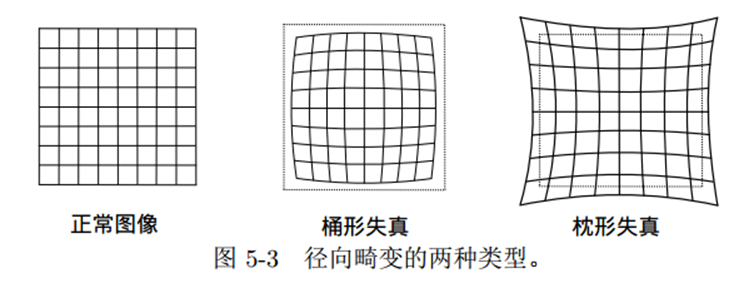桶形畸变是由于图像放大率随着离光轴的距离增加而减小，而枕形畸变却恰好相反。
在这两种畸变中，穿过图像中心和光轴有交点的直线还能保持形状不变。

除了透镜的形状会引入径向畸变外，在相机的组装过程中由于不能使得透镜和成像面严格平行也会引入切向畸变。

平面上的任意一点 p p 可以用笛卡尔坐标表示为 [ x , y ] T [x, y]^{T} , 也可以把它写成极坐标的形式
[ r ; θ ] T [r; θ]^T ，其中 r r 表示点 p p 离坐标系原点的距离， θ θ 表示和水平轴的夹角。径向畸变可看成坐标点沿着长度方向发生了变化 δ r δr , 也就是其距离原点的长度发生了变化。切向畸变可以
看成坐标点沿着切线方向发生了变化，也就是水平夹角发生了变化 δ θ δθ

对于径向畸变，无论是桶形畸变还是枕形畸变，由于它们都是随着离中心的距离增加
而增加。我们可以用一个多项式函数来描述畸变前后的坐标变化：这类畸变可以用和距中
心距离有关的二次及高次多项式函数进行纠正：

x corrected = x ( 1 + k 1 r 2 + k 2 r 4 + k 3 r 6 ) y corrected = y ( 1 + k 1 r 2 + k 2 r 4 + k 3 r 6 ) \begin{array}{l}{x_{\text {corrected}}=x\left(1+k_{1} r^{2}+k_{2} r^{4}+k_{3} r^{6}\right)} \\ {y_{\text {corrected}}=y\left(1+k_{1} r^{2}+k_{2} r^{4}+k_{3} r^{6}\right)}\end{array}

其中 [ x , y ] T [x, y]^{T} 是未纠正的点的坐标， [ x c o r r e c t e d ; y c o r r e c t e d ] T [xcorrected; ycorrected]^T 是纠正后的点的坐标，注意它们都是归一化平面上的点，而不是像素平面上的点。在上式中，对于畸变较小的图像中心区域，畸变纠正主要是 k 1 k1 起作用。而对于畸变较大的边缘区域主要是 k 2 k2 起作用。普通摄像头用这两个系数就能很好的纠正径向畸变。对畸变很大的摄像头，比如鱼眼镜头，可以加入 k 3 k3 畸变项对畸变进行纠正。

另一方面，对于切向畸变，可以使用另外的两个参数 p 1 , p 2 p1, p2 来进行纠正:

x corrected = x + 2 p 1 x y + p 2 ( r 2 + 2 x 2 ) y corrected = y + p 1 ( r 2 + 2 y 2 ) + 2 p 2 x y \begin{array}{l}{x_{\text {corrected}}=x+2 p_{1} x y+p_{2}\left(r^{2}+2 x^{2}\right)} \\ {y_{\text {corrected}}=y+p_{1}\left(r^{2}+2 y^{2}\right)+2 p_{2} x y}\end{array}

对于相机坐标系中的一点 P ( X ; Y ; Z ) P(X; Y; Z) ，我们能够通过五个畸变系数找到这个点在像素平面上的正确位置:

1. 将三维空间点投影到归一化图像平面。设它的归一化坐标为 [x; y]T。
2. 对归一化平面上的点进行径向畸变和切向畸变纠正。
3. 将纠正后的点通过内参数矩阵投影到像素平面，得到该点在图像上的正确位置。
1. 首先，世界坐标系下有一个固定的点 P_w；
2. 由于相机在运动，它的运动由 R; t 或变换矩阵描述。 P 的相机坐标为：
P_c= R * P_w + t。
3. 这时的 P_c 仍有 X; Y; Z 三个量，把它们投影到归一化平面 Z = 1 上，得到 P 的归
一化相机坐标： Pc = [X/Z; Y /Z; 1]T 。
4. 最后, P 的归一化坐标经过内参后，对应到它的像素坐标： P_uv = K * P_c。

双目坐标

1.理想情况的双目相机模型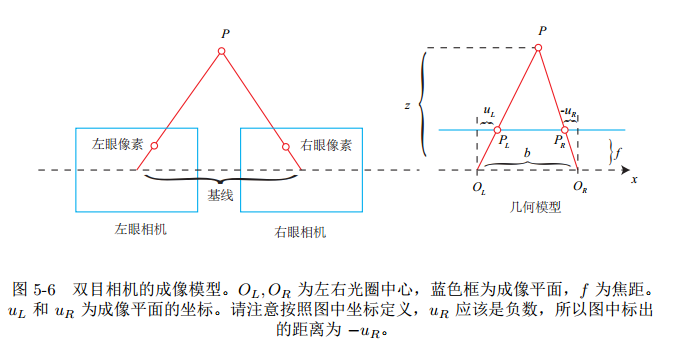z − f z = b − u l + u r b \frac{z-f}{z}=\frac{b-u_l+u_r}{b} , 因为 d = u l − u r d=u_l-u_r ,
所以最后 z = f ∗ b d z=\frac{f*b}{d}

2. 真实的双目相机模型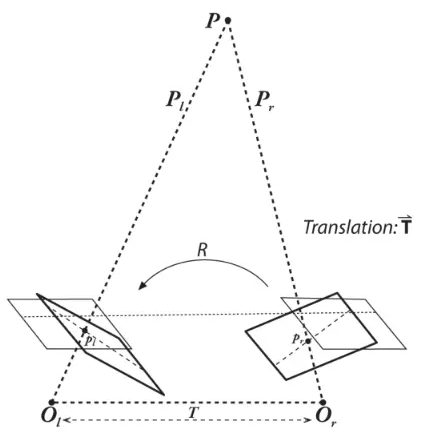真实场景的双目摄像机其实不是严格平行向前。所以需要双目标定得到两个相机之间的平移和旋转矩阵
参考：《视觉slam十四讲》

展开全文qq_33278461 2019-09-19 16:12:21
• 基座坐标系是描述机器人TCP在三维空间运动所必需的基本坐标系，机器人的手动操作、程序自动运行、加工作业都离不开基座坐标系，因此，任何机器人都需要有基座坐标系。 基座坐标系通常以腰回转轴线为Z轴、以机器人...

想学好工业机器人就要熟悉机器人的控制，工业机器人基本靠控制运动轴位置和电磁元件通断来进行运动。运动轴位置控制一般用来改变工具作业点，它可驱动机器人关节运动或机器人、工件的整体移动；在机器人应用程序中，运动轴位置可通过基本移动指令控制。基本移动指令属于通用指令，只要机器人的控制系统相同，即使工业机器人的用途有所区别，但移动指令的格式、编程要求仍一致。

部分工业机器人可能还包含特殊的运动轴位置控制要求，例如，点焊机器人的伺服焊钳控制、分拣机器人的同步跟踪控制、探测机器人的传感器引导运动和摄像控制等。此类机器人的控制系统通常需要选配特殊功能，并通过专门的RAPID移动指令进行控制。

多关节机器人的运动复杂，为了实现对机器人本体运动轴的控制，需要对机器人的工具参考点(Tool Reference Point，TRP)位置进行定义，以便建立其运动控制模型。

例如，在图示的6轴串联机器人上，TRP就是机器人手腕上的工具安装法兰中心点。为了确定TRP，在控制系统中，需要定义基座高度(height_of_foot)，j2、j3轴中心偏移(offset_off_joint_2、offset_off_joint_3)，下臂、上臂长度(length_of_lower_arm、length_of_upper_arm)以及手腕长度(length_of_wrist)等结构参数。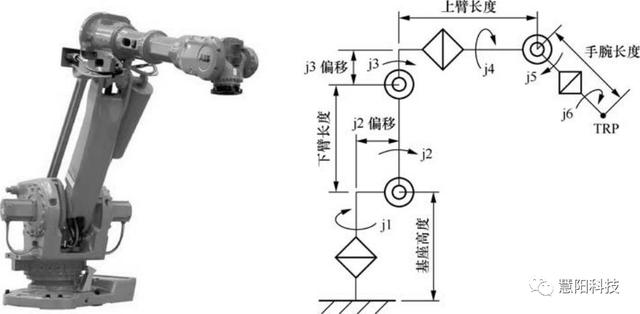结构参数是机器人运动控制的前提条件，且与机器人结构密切相关，故需要机器人生产厂家在系统参数上设置。在RAPID应用程序中，ABB机器人的结构参数一般在系统模块(System module)中定义，该模块由ABB公司编制，并可在系统启动时自动加载，并且不受用户作业程序删除操作的影响。

TRP也是确定工具作业点、设定工具数据的基准位置。工业机器人的工具作业点又称为工具控制点(Tool Control Point，TCP)或工具中心点(Tool Center Point，TCP)，它是机器人关节、直线、圆弧插补等移动指令的控制对象，指令中的起点、终点就是TCP在指定坐标系上的位置值。TCP的位置与工具形状、安装方式密切相关；不安装工具时，TCP就是TRP。

机器人作业程序中的移动指令大多用来控制机器人TCP和工件(或基准)的相对运动， TCP的位置取决于机械部件的运动。例如，通过机器人本体的运动，可使TCP和机器人基座产生相对运动；通过机器人基座的整体移动，可使TCP和大地产生相对运动；通过工件的整体运动，可使TCP和工件产生相对运动等。

可驱动机器人TCP运动的运动轴众多、组成形式多样，简单系统可能只有单一的机器人本体运动；复杂机系统可能需要控制多个机器人以及机器人变位器、工件变位器等诸多辅助部件运动。例如，下图所示的双机器人协同作业系统，实际上包含机器人1、机器人2、机器人变位器、工件变位器共4个运动部件。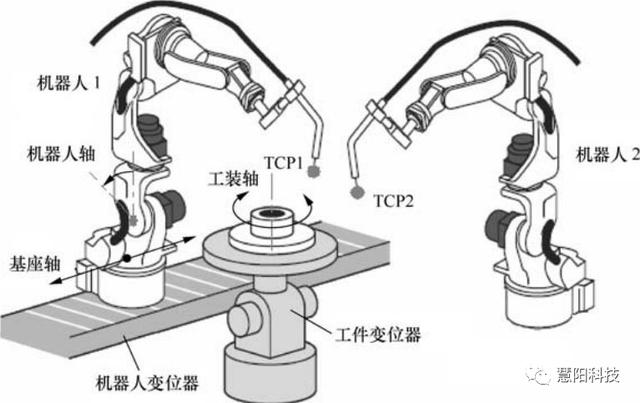为了便于控制与编程，在控制系统内部，通常需要根据机械部件的功能与用途，对运动轴进行分组管理，将系统分为若干个具有独立功能和若干个运动轴的基本运动单元。在ABB机器人说明书中，将这样的单元称为机械单元(Mechanical unit)；而在安川等公司生产的机器人说明书中，则将其称为“控制轴组”。

工业机器人系统的运动轴一般可分为机器人单元(机器人轴组)、基座单元(基座轴组)和工装单元(工装轴组)3类。

01机器人单元

机器人轴是用于机器人本体运动控制的坐标轴，用来控制机器人TCP和机器人基座的相对运动。在多机器人控制系统上，可分为机器人1(ROB_1)、机器人2 (ROB_2)等多个轴组(单元)；机器人单元一旦选定，对应的机器人就成为系统的控制对象。

02基座单元

基座轴是驱动机器人安装基座、实现机器人整体变位的辅助运动轴，用来控制机器人基座及TCP和大地的相对运动。基座单元一旦选定，机器人变位器就成为系统的控制对象。

03工装单元

工装轴是驱动工装运动、实现工件整体变位的辅助运动轴，用来控制机器人TCP和工件的相对运动。工装单元一旦选定，工件变位器就成为系统的控制对象。

机器人轴是系统的基本运动轴，任何工业机器人都具备；基座轴、工装轴是用于机器人、工件整体移动的辅助轴，当系统配置有变位器时需要使用。在工业机器人系统中，基座轴、工装轴统称为“外部轴”或“外部关节”(extjoint)。

同一机械单元的所有运动轴可进行成组管理。机器人操作或编程时，可根据需要，通过生效/撤销机械单元来改变系统的控制对象。

在ABB机器人中，机械单元(控制轴组)可通过系统参数定义，不同的机械单元需要定义不同的名称。在RAPID程序中，可通过机械单元启用/停用指令，来使能/关闭指定机械单元全部运动轴的伺服驱动器，使该机械单元的运动轴处于实时位置控制状态，或处于保持位置不变的伺服锁定状态。

机器人TCP的运动需要通过三维笛卡尔直角坐标系来描述。在ABB机器人中，笛卡尔坐标系有下图所示的大地坐标系(World coordinates)、基座坐标系(Base coordinates)、工具坐标系(Tool coordinates)、用户坐标系(User coordinates)和工件坐标系(Object coordinates)等，说明如下。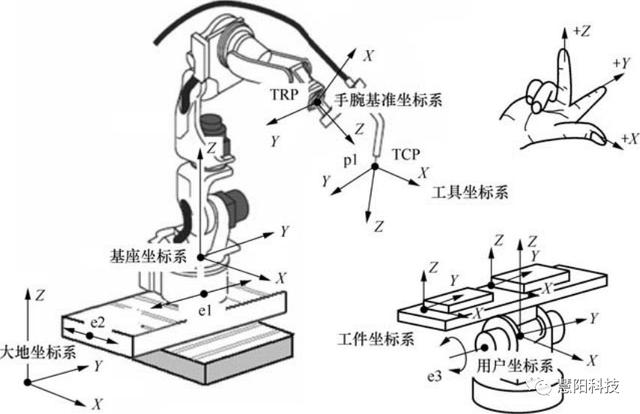大地坐标系

大地坐标系(World coordinates)有时被译为“世界坐标系”，它是以地面为基准的三维笛卡尔直角坐标系，可用来描述物体相对于地面的运动。在多机器人协同作业系统或使用机器人变位器的系统中，为了确定机器人(基座)的位置，需要建立大地坐标系。此外，如机器人采用了下图所示的倒置或倾斜安装，大地坐标系也是设定基座坐标系的基准。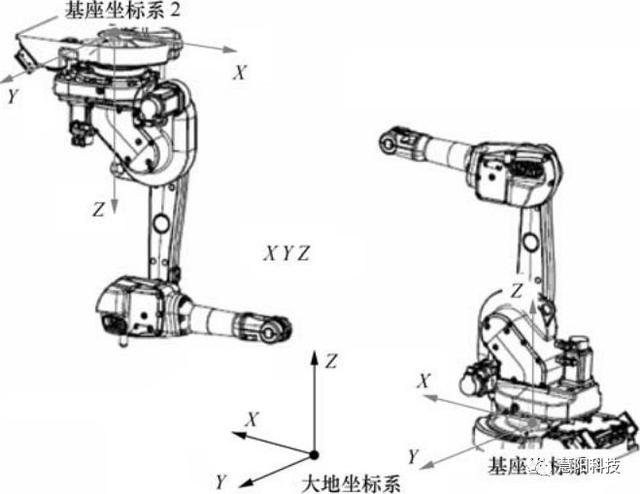通常情况下，地面垂直安装的机器人基座坐标系与大地坐标系的方向一致，因此，对于常用的、地面垂直安装的单机器人系统，系统默认为大地坐标系和基座坐标系重合，此时，可不设定大地坐标系。

基座坐标系

基座坐标系(Base coordinates)亦称为机器人坐标系，它是以机器人安装基座为基准、用来描述机器人本体运动的虚拟笛卡尔直角坐标系。基座坐标系是描述机器人TCP在三维空间运动所必需的基本坐标系，机器人的手动操作、程序自动运行、加工作业都离不开基座坐标系，因此，任何机器人都需要有基座坐标系。

基座坐标系通常以腰回转轴线为Z轴、以机器人安装底面为XY平面；其Z轴正向与腰回转轴线方向相同，X轴轴线与腰回转轴j1的0°线重合，手腕离开机器人向外方向为X轴正向；Y轴由图3.1-3所示的右手定则确定。

工具坐标系

工具坐标系(Tool coordinates)是机器人作业必需的坐标系，建立工具坐标系的目的是确定工具的TCP位置和安装方式(姿态)。通过建立工具坐标系，机器人使用不同的工具作业时，只需要改变工具坐标系(工具数据tooldata)，就能保证TCP到达指令点，而无需对程序进行其他修改。

机器人手腕上的工具安装法兰面和中心点是工具的安装定位基准。以工具安装法兰中心点(TRP)为原点、垂直工具安装法兰面向外的方向为Z轴正向、手腕向机器人外侧运动的方向为X轴正向的虚拟笛卡尔直角坐标系，称为机器人的手腕基准坐标系。手腕基准坐标系是建立工具坐标系的基准，如未设定工具坐标系，控制系统将默认为工具坐标系和手腕基准坐标系重合。

工具坐标系是用来确定工具TCP位置和工具方向(姿态)的坐标系，它通常是以TCP为原点、以工具接近工件方向为Z轴正向的虚拟笛卡尔直角坐标系；常用的点焊、弧焊机器人的工具坐标系一般如下图所示。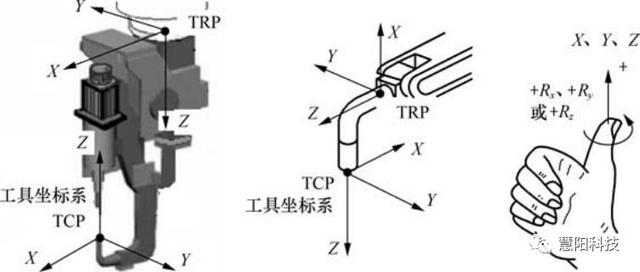用户坐标系

用户坐标系(User coordinates)是以下图所示的工装位置为基准来描述TCP运动的虚拟笛卡尔直角坐标系，通常用于工装移动协同作业系统或多工位作业系统。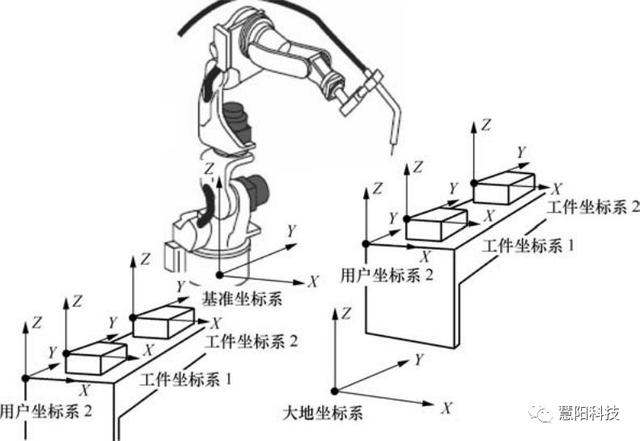通过建立用户坐标系，机器人在不同工位进行相同作业时，只需要改变用户坐标系(工件数据wobjdata)，就能保证工具TCP到达指令点，而无需对程序进行其他修改。

在RAPID程序中，用户坐标系可通过工件数据(wobjdata)定义，有关内容详见后述。对于通常的工件固定、机器人移动工具作业，用户坐标系以大地坐标系为基准建立；对于工具固定、机器人移动工件作业，用户坐标系则以手腕基准坐标系为基准建立。

工件坐标系

工件坐标系(Object coordinates)是以工件为基准来描述TCP运动的虚拟笛卡尔坐标系。通过建立工件坐标系，机器人需要对不同工件进行相同作业时，只需要改变工件坐标系，就能保证工具TCP到达指令点，而无需对程序进行其他修改。

工件坐标系可在用户坐标系的基础上建立，并允许有多个。对于工具固定、机器人用于工件移动的作业，必须通过工件坐标系来描述TCP与工件的相对运动。

在RAPID程序中，工件坐标系同样需要通过工件数据(wobjdata)定义；如果机器人仅用于单工件作业，系统默认用户坐标系和工件坐标系重合，无需另行设定工件坐标系。

本期主题的选择来自于对产品升级规划时的思考，我们计划在机器人基础教学领域打造精品，所以选择了一些优秀的专业资料进行参考，我们希望通过我们对知识的加工处理让这些稍显枯燥的文字变得生动有趣，让机器人教学变得简单，所以您一定有机会在后面的专题中看到我们对这些知识的重新解读。本期内容全部引自《ABB工业机器人编程全集》一书，特此说明。

展开全文weixin_39860757 2020-11-21 04:22:35
• 立式加工中心的坐标系是什么？有多少人和我有同样的疑惑，记得初步了解加工中心的时候这个问题，我是真的分不清，只能知道的是立式加工中心它有三条轴：X轴Y轴Z轴。但是加工中心的操机人员或者是编程人员的话，这样...

立式加工中心的坐标系是什么？有多少人和我有同样的疑惑，记得初步了解加工中心的时候这个问题，我是真的分不清，只能知道的是立式加工中心它有三条轴：X轴Y轴Z轴。但是加工中心的操机人员或者是编程人员的话，这样浅显的了解是不够的。因为如果对于坐标系的概念模糊，分不清状况的话，是和容易在操机或者是编程中出现失误的。为了能够对加工中心的坐标系更加深入详细的了解，我在网上搜罗了许多的相关资料信息，在下文中我们详细的罗列出来坐标系的相关内容，有需要的朋友可以详细查看一下：经过上文的分析，相信大家对于加工中心的坐标系都有了一个比较清楚的了解，
本文出自依速力机械原创，转载请注明出处：http://www.yisuli.com/Article/lsjgzxzbxs_1.html

转载于:https://blog.51cto.com/13504147/2045164

展开全文weixin_33929309 2017-11-28 16:03:30
• 左手坐标系与右手坐标系 矩阵

qq_42564908 2020-07-23 10:59:38
• 大隈机床坐标系调整方法 坐标系

21KB mglinhaixueyuan 2013-01-03 11:03:36
• 605KB woshifafuge 2021-10-08 07:19:14
• UG NX 12 坐标系 UG NX

weixin_43911798 2021-09-22 10:08:39
• qq_30722721 2019-08-12 16:56:11
• qq_35250841 2020-05-18 18:57:00
• zhangjunhit 2019-05-30 11:33:05
• 4.56MB huangjiuchao 2011-09-28 10:15:56
• python绘制笛卡尔直角坐标系 笛卡尔直角坐标系 matplotlib python

zengbowengood 2019-11-01 17:45:03
• 数控加工程序编制数控车1建立工件坐标系.pptx 技术

5.87MB xiaowu198809 2021-10-09 00:19:53
• 295KB m0_52957036 2020-10-17 10:49:23
• weixin_42509614 2021-01-14 05:06:54
• 502KB weixin_38618312 2020-07-02 12:49:57
• weixin_39694174 2020-11-16 13:11:32
• weixin_42543451 2019-06-09 18:19:53
• weixin_43911798 2020-09-07 16:29:03
• weixin_43911798 2021-03-30 08:35:52
• UG NX 12 坐标系的操作 UG NX

weixin_43911798 2021-11-04 15:26:10
• 321KB programcx 2021-09-12 18:34:56
• weixin_43911798 2020-09-01 09:40:15
• weixin_43911798 2021-06-02 13:40:47...